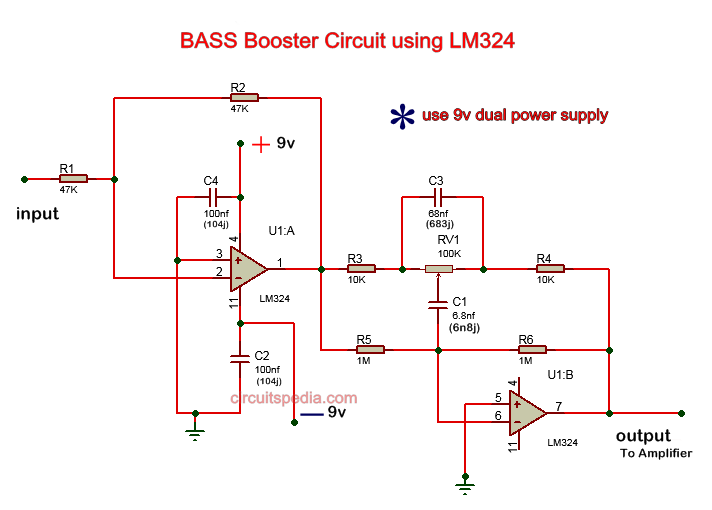Turbo bass circuit

Bass booster circuit diagram

This bass booster circuit is made using the quad op-amp ic LM324. This op-amp ic has  4 operational amplifiers, but in this circuit, only 2 op-amps are used. This circuit provides Low pass filter of input audio which produces a Low frequency of input and high frequency is blocked.

Connect the circuit as the given diagram as below . Give input through the 47K resistor at pin no 2, one other 47 K resistor is connected between the pin 1 and pin 2. Pin 1 is the output pin of 1st opamp and this output pin is connected with 2nd op-amp input inverting pin 6 through resistors and capacitors. Pin no 4 is connected with +9v supply. pin 11 is connected with -9v and connect the ground with given terminals in diagram. Use dual power supply for the operation of this circuit.RV1 is 100k preset for adjusting the level of frequency. Pin no 7 is the output of 2nd opamp and this pin is used in this circuit as the output also. Output must be connected to the amplifier input.

Schematic diagramdual power supply Schematic diagram